# 【RL-GAN-Net】强化学习控制GAN网络，用于实时点云形状的补全。

• 论文题目RL-GAN-Net: A Reinforcement Learning Agent Controlled GAN Network for Real-Time Point Cloud Shape Completion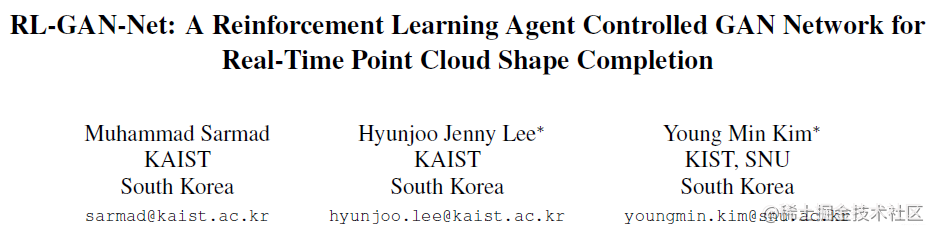## 所解决的问题

用强化学习控制GAN网络，以使得GAN更快，更鲁棒。将其用于点云数据生成。全网第一次用RL控制GAN。通过数据驱动的方法填补三维数据中的数据缺失。

## 所采用的方法？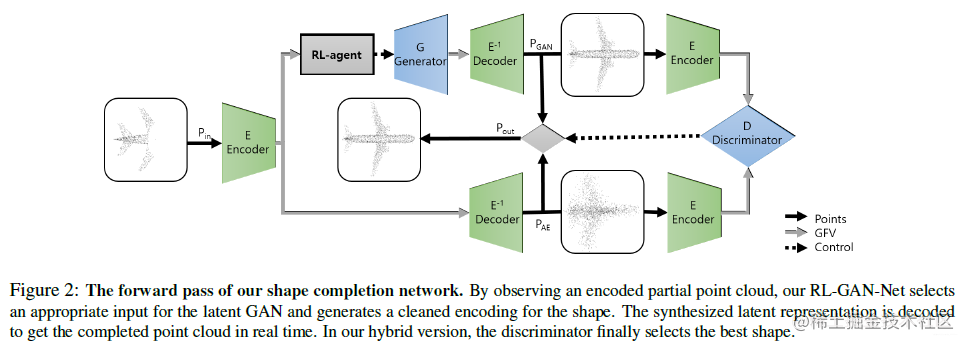预训练阶段，训练一个自编码器，用于生成隐空间的表示，之后用这个去训练GAN网络。强化学习智能体用于选择合适的$z$向量，去合成隐空间的表示。与之前的反向传播发现$z$向量不同，本文采用RL的方法进行选择。

主要由三个模块组成：1. 自编码器；2. $l$-GAN；3. 强化学习智能体(RL)。

#### 自编码器

自编码器用的损失函数如下：

$d_{C H}\left(P_{1}, P_{2}\right)=\sum_{a \in P_{1}} \min _{b \in P_{2}}\|a-b\|_{2}^{2}+\sum_{b \in P_{2}} \min _{a \in P_{1}}\|a-b\|_{2}^{2}$

其中$P_{1}$$P_{2}$代表点云的输入和输出。

#### $l$-GAN

结合GFV来训练GAN

• Chamfer loss:

输入点云数据$P_{in}$和生成器和解码器输出数据$E^{-1}(G(z))$loss

$L_{C H}=d_{C H}\left(P_{i n}, E^{-1}(G(z))\right)$

• GFV loss：生成CFV $G(z)$和输入点云$E(P_{in})$

$L_{G F V}=\left\|G(z)-E\left(P_{i n}\right)\right\|_{2}^{2}$

• Discriminator loss 判别器损失函数：

$L_{D}=-D(G(z))$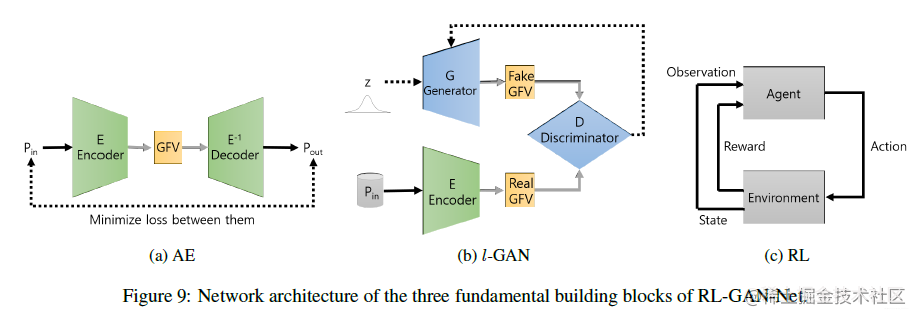#### 强化学习

强化学习用于快速选择GAN生成器的输入$z$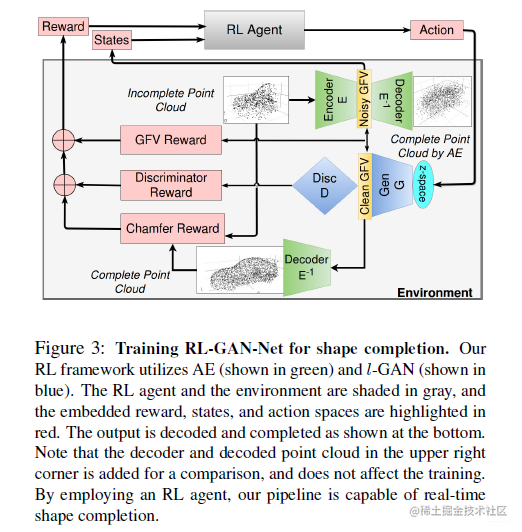奖励函数定义为：

$r=w_{C H} \cdot r_{C H}+w_{G F V} \cdot r_{G F V}+w_{D} \cdot r_{D}$

其中 $r_{CH}=-L_{CH}$$r_{GFV}=-l_{GFV}$$r_{D}=-L_{D}$。智能体用DDPG算法。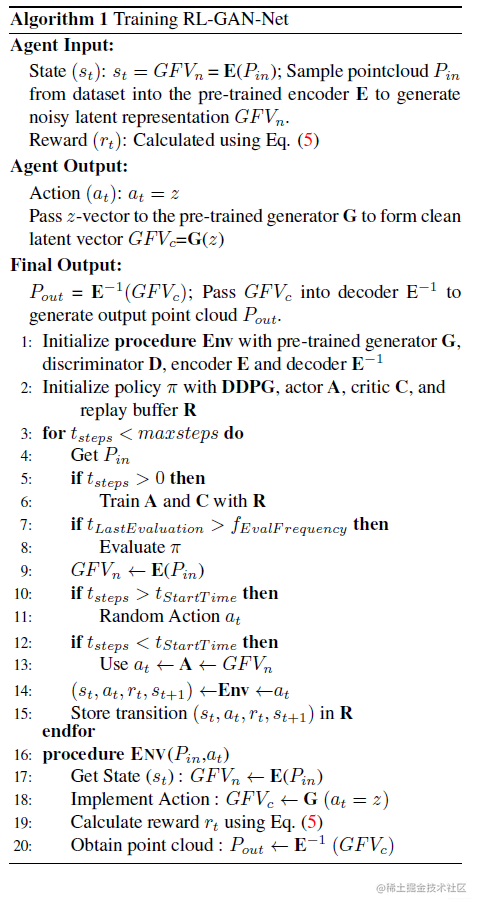## 取得的效果？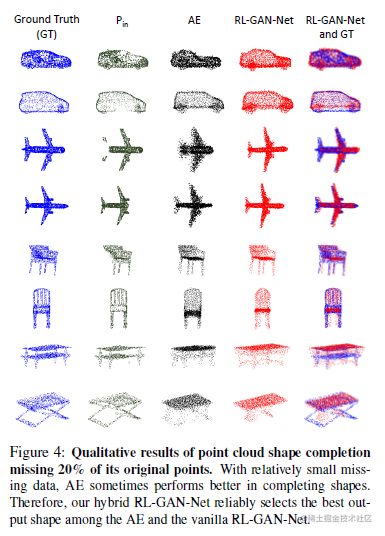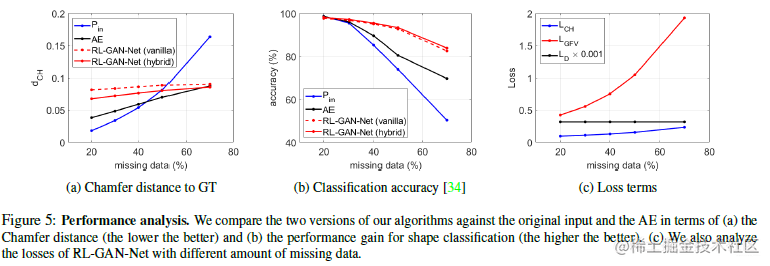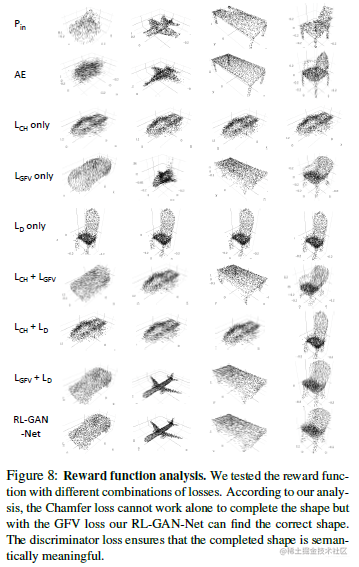## 参考资料

相似文献

• Panos Achlioptas, Olga Diamanti, Ioannis Mitliagkas, and Leonidas J. Guibas. Representation learning and adversarial generation of 3d point clouds. CoRR, abs/1707.02392, 2017. (有提到用隐空间数据训练GAN会更稳定)。

相关GitHub链接